# modwtxcorr

Wavelet cross-correlation sequence estimates using the maximal overlap discrete wavelet transform (MODWT)

## Syntax

``xcseq = modwtxcorr(w1,w2)``
``xcseq = modwtxcorr(w1,w2,wav)``
``````[xcseq,xcseqci] = modwtxcorr(___)``````
``````[xcseq,xcseqci] = modwtxcorr(w1,w2,wav,conflevel)``````
``````[xcseq,xcseqci,lags] = modwtxcorr(___)``````
``[___] = modwtxcorr(___,'reflection')``

## Description

example

````xcseq = modwtxcorr(w1,w2)` returns the wavelet cross-correlation sequence estimates for the maximal overlap discrete wavelet transform (MODWT) transforms specified in `w1` and `w2`. `xcseq` is a cell array of vectors where the elements in each cell correspond to cross-correlation sequence estimates. If there are enough nonboundary coefficients at the final level, `modwtxcorr` returns the scaling cross-correlation sequence estimate in the final cell of `xcseq`. ```

example

````xcseq = modwtxcorr(w1,w2,wav)` uses the wavelet `wav` to determine the number of boundary coefficients by level.```

example

``````[xcseq,xcseqci] = modwtxcorr(___)``` returns in `xcseqci` the 95% confidence intervals for the cross-correlation sequence estimates in `xcseq`, using any arguments from the previous syntaxes. ```

example

``````[xcseq,xcseqci] = modwtxcorr(w1,w2,wav,conflevel)``` uses `conflevel` for the coverage probability of the confidence interval. `conflevel` is a real scalar strictly greater than 0 and less than 1. If `conflevel` is unspecified or specified as empty, the coverage probability defaults to 0.95. ```

example

``````[xcseq,xcseqci,lags] = modwtxcorr(___)``` returns the lags for the wavelet cross-correlation sequence estimates in a cell array of column vectors. ```

example

````[___] = modwtxcorr(___,'reflection')` reduces the number of wavelet and scaling coefficients at each scale by half before computing the cross-correlation sequences. Specifying the `'reflection'` option in `modwtxcorr` is equivalent to first obtaining the MODWT of `w1` `w2` with `'periodic'` boundary handling and then computing the wavelet cross-correlation sequence estimates. Use this option only when you obtain the MODWT of `w1` and `w2` using the `'reflection'` boundary condition. You must enter the entire character vector `'reflection'`. If you added a wavelet named `'reflection'` using the wavelet manager, you must rename that wavelet prior to using this option. `'reflection'` may be placed in any position in the input argument list after the input transforms `w1` `w2`. ```

## Examples

collapse all

Obtain the MODWT of the Southern Oscillation Index and Truk Islands pressure data. The sampling period is one day.

```load soi load truk wsoi = modwt(soi); wtruk = modwt(truk);```

Compute the wavelet cross-correlation sequences. Examine the level-five cross-correlation sequence corresponding to a scale of 32-64 days. Determine the index corresponding to a lag of zero and plot out to 240 lags.

```xcseq = modwtxcorr(wsoi,wtruk); zerolag = floor(numel(xcseq{5})/2)+1; plot(xcseq{5}(zerolag:zerolag+240),'linewidth',2) set(gca,'xlim',[1 240]); title({'Cross-Correlation Sequence Level 5'; 'Scale: 32-64 Days'}); hold off```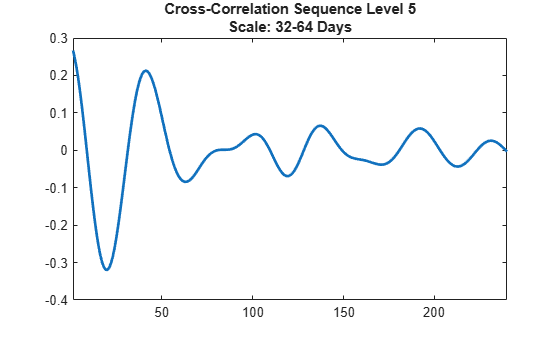Obtain the MODWT of the Southern Oscillation Index and Truk Islands pressure data using the Fejér-Korovkin wavelet filter with 8 coefficients. The sampling period of the data is one day.

```load soi load truk wsoi = modwt(soi,'fk8'); wtruk = modwt(truk,'fk8');```

Compute the wavelet cross-correlation sequences. Examine the level-five cross-correlation sequence corresponding to a scale of 32-64 days. Determine the index corresponding to a lag of zero and plot out to 240 lags.

```xcseq = modwtxcorr(wsoi,wtruk,'fk8'); zerolag = floor(numel(xcseq{5})/2)+1; plot(xcseq{5}(zerolag:zerolag+240),'linewidth',2) set(gca,'xlim',[1 240]); title({'Cross-Correlation Sequence Level 5'; 'Scale: 32-64 Days'}); hold off```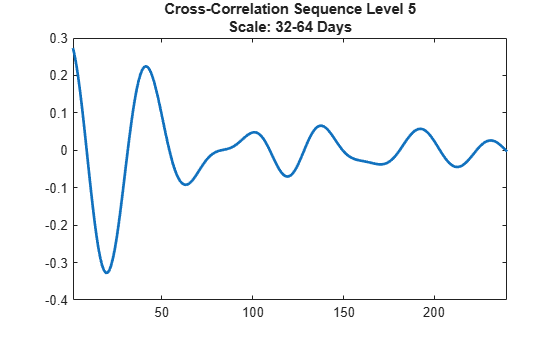Plot the wavelet cross-correlation with 95% confidence intervals at scale 4 for two 5-Hz sine wave signals with additive noise.

```dt = 0.01; t = 0:dt:6; x = cos(2*pi*5*t)+1.5*randn(size(t)); y = cos(2*pi*5*t-pi)+2*randn(size(t)); wx = modwt(x,'fk14',5); wy = modwt(y,'fk14',5); modwtcorr(wx,wy,'fk14') j = 4; [xcseq,xcseqci] = modwtxcorr(wx,wy,'fk14'); zerolag = floor(numel(xcseq{j})/2)+1; lagidx = zerolag-30:zerolag+30; plot(xcseq{j}(lagidx)); hold on; grid plot(xcseqci{j}(lagidx,:),'r--'); xlabel('Samples'); title('Scale: 0.32-0.16 Seconds'); ```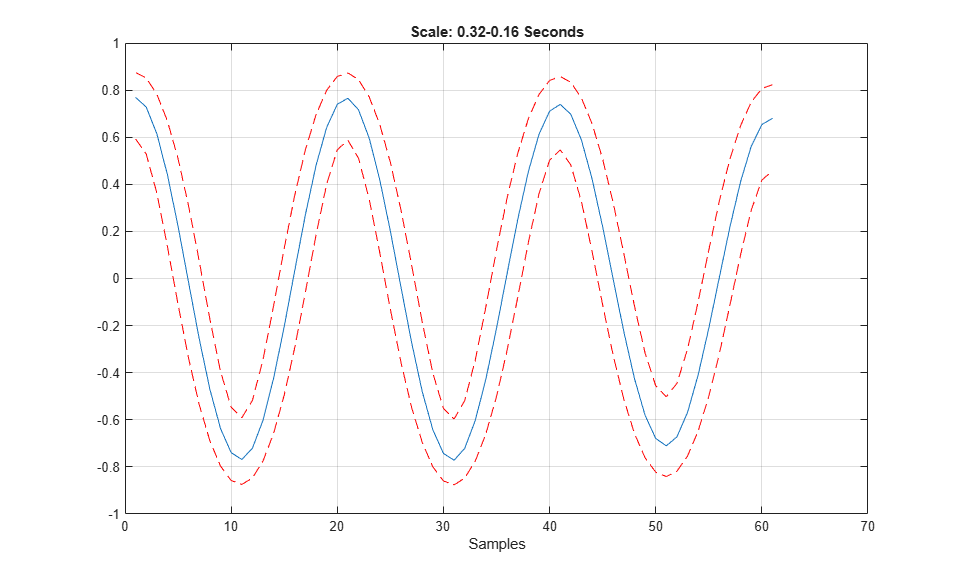Compare the .90 and .95 (default) confidence intervals for the wavelet cross-correlation at level four.

Obtain the MODWT for two noisy sine waves using the Fejér-Korovkin with 14 coefficients, and specify the level to use.

```dt = 0.01; t = 0:dt:6; x = cos(2*pi*5*t)+1.5*randn(size(t)); y = cos(2*pi*5*t-pi)+2*randn(size(t)); wx = modwt(x,'fk14',4); wy = modwt(y,'fk14',4); lev = 4; [xcseq,xcseqci] = modwtxcorr(wx,wy,'fk14'); [xcseq90,xcseqci90] = modwtxcorr(wx,wy,'fk14',0.90); zerolag = floor(numel(xcseq{lev})/2)+1; zerolag90 = floor(numel(xcseq90{lev})/2)+1; lagidx = zerolag-30:zerolag+30; lagidx90 = zerolag90-30:zerolag90+30; plot(xcseqci{lev}(lagidx,:),'--r'); hold on plot(xcseqci90{lev}(lagidx90,:),'--b'); plot(xcseq{lev}(lagidx),'-k','LineWidth',1); grid title('.90 and .95 Confidence Levels')```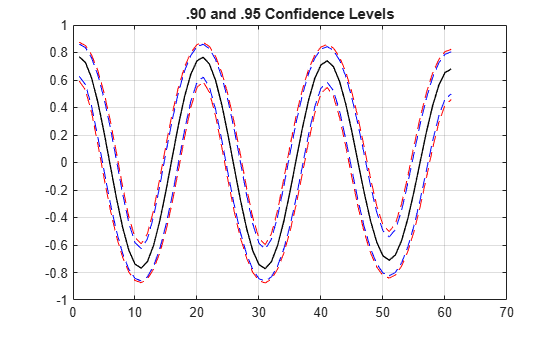Notice that the .95 confidence interval width (in red) is larger than the .90 confidence interval width (in blue).

Plot the cross-correlation sequence estimate for the Southern Oscillation Index and Truk Island pressure data. Estimate 95% confidence intervals for scale of ${2}^{5}$ days.

```load soi load truk wsoi = modwt(soi); wtruk = modwt(truk); [xcseq,xcseqci,lags] = modwtxcorr(wsoi,wtruk); plot(lags{5},xcseq{5},'linewidth',2) hold on plot(lags{5},xcseqci{5},'r--') set(gca,'xlim',[-120 120]); xlabel('Lag (Days)'); grid title({'Cross-Correlation Sequence Level 5'; 'Scale: 32-64 Days'}); hold off```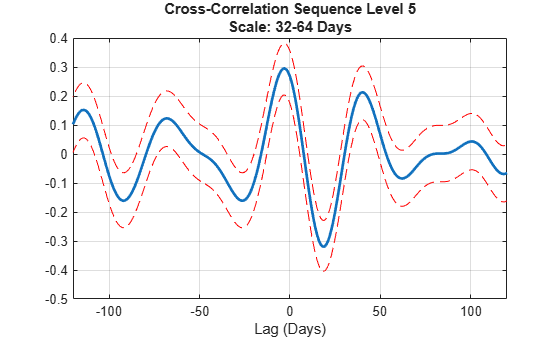Obtain the MODWT of 36 years of Southern Oscillation Index and Truk Islands pressure data with both periodic and reflection boundary conditions. The `modwt` function with the `'reflection'` option extends the input signal symmetrically at the right boundary. The input signal is then twice its original length. MODWTXCORR with the reflection boundary handling reduces the number of wavelet and scaling coefficients at each half before computing the cross-correlation sequences. The size of the cross-correlation sequences is the same as acquiring the MODWT with the default periodic boundary condition.

```load soi load truk```

Obtain the MODWT with the default periodic boundary condition.

```wsoi_default = modwt(soi); wtruk_default = modwt(truk);```

Obtain the MODWT with the reflection boundary condition.

```wsoi_reflect = modwt(soi,'reflection'); wtruk_reflect = modwt(truk,'reflection');```

Obtain the cross-correlation sequences.

```xcseq_default = modwtxcorr(wsoi_default,wtruk_default); xcseq_reflect = modwtxcorr(wsoi_reflect,wtruk_reflect,'reflection');```

Compare the number of elements in the cell array output for both boundary conditions.

`cellfun(@numel,xcseq_reflect)`
```ans = 10×1 25981 25953 25897 25785 25561 25113 24217 22425 18841 11673 ```
`cellfun(@numel,xcseq_default)`
```ans = 10×1 25981 25953 25897 25785 25561 25113 24217 22425 18841 11673 ```

## Input Arguments

collapse all

MODWT transform of signal 1, specified as a matrix of doubles. The input `w1` must be the same size as `w2` and must have been obtained with the same wavelet. By default, `modwtxcorr` assumes that you obtained the MODWT using the symlet wavelet with four vanishing moments, `'sym4'`).

MODWT transform of signal 2, specified as a matrix of doubles. The input `w2` must be the same size as `w1` and must have been obtained with the same wavelet. By default, `modwtxcorr` assumes that you obtained the MODWT using the symlet wavelet with four vanishing moments (`'sym4'`).

Wavelet, specified as a character vector or string scalar, indicating a valid wavelet, or as a positive even integer indicating the length of the wavelet and scaling filters. If `wav` is unspecified or specified as an empty, `[]`, `wav` defaults to `'sym4'`.

Confidence level, specified as a positive scalar less than 1. `conflevel` determines the coverage probability of the confidence intervals in `xcseqci`. If unspecified, or specified as empty, `[]`, `conflevel` defaults to 0.95.

## Output Arguments

collapse all

Cross-correlation sequences by scale, returned as a cell array of vectors. The vectors are of size 2NJ-by-1, where NJ is the number of nonboundary coefficients by level (scale). This level is the minimum of `size(w1,1)` and `floor(log2(N/(L-1)+1))` where N is the length of the data and L is the filter length. If there are enough nonboundary coefficients at the final level, `modwtxcorr` returns the scaling cross-correlation sequence estimate in the final cell of `xcseq`.

Confidence intervals by scale, returned as a cell array of matrices. The size of each matrix is (2NJ-1)-by-2, where NJ is the number of nonboundary coefficients by level (scale).

• For the .95 default value, the first column of the ith element of `xcseqci` contains the lower 95% confidence bound for the cross-correlation coefficient at each lag.

• For the .95 default value, the second column contains the upper 95% confidence bound.

Confidence bounds are computed using Fisher's Z-transformation. The standard error of Fisher's Z statistic is the square root of N–3. In this case, N is the equivalent number of coefficients in the critically sampled discrete wavelet transform (DWT), `floor(size(w1,2)/2^LEV)`, where `LEV` is the level of the wavelet transform. `modwtcorr` returns NaNs for the confidence bounds when N-3 is less than or equal to zero.

Lags for the cross-correlation sequences, returned as a cell array of vectors. `lags` is a cell array of column vectors the same length as `xcseq`. The elements in each cell of `xcseq` correspond to the cross-correlation sequence estimates at lags from -(NJ-1) to (NJ-1), where NJ is the number of nonboundary coefficients at level J. The 0th lag element is located at the index floor((2*NJ-1)/2)+1.

 Percival, D. B., and A. T. Walden. Wavelet Methods for Time Series Analysis. Cambridge, UK: Cambridge University Press, 2000.

 Whitcher, B., P. Guttorp, and D. B. Percival. "Wavelet analysis of covariance with application to atmospheric time series." Journal of Geophysical Research, Vol. 105, 2000, pp. 14941–14962.